CBSE Class 12 Sample Paper for 2018 Boards

Class 12
Solutions of Sample Papers and Past Year Papers - for Class 12 Boards

### Find ∫ sec⁡ x /(1 + cosec x)  dx

This is a question of CBSE Sample Paper - Class 12 - 2017/18.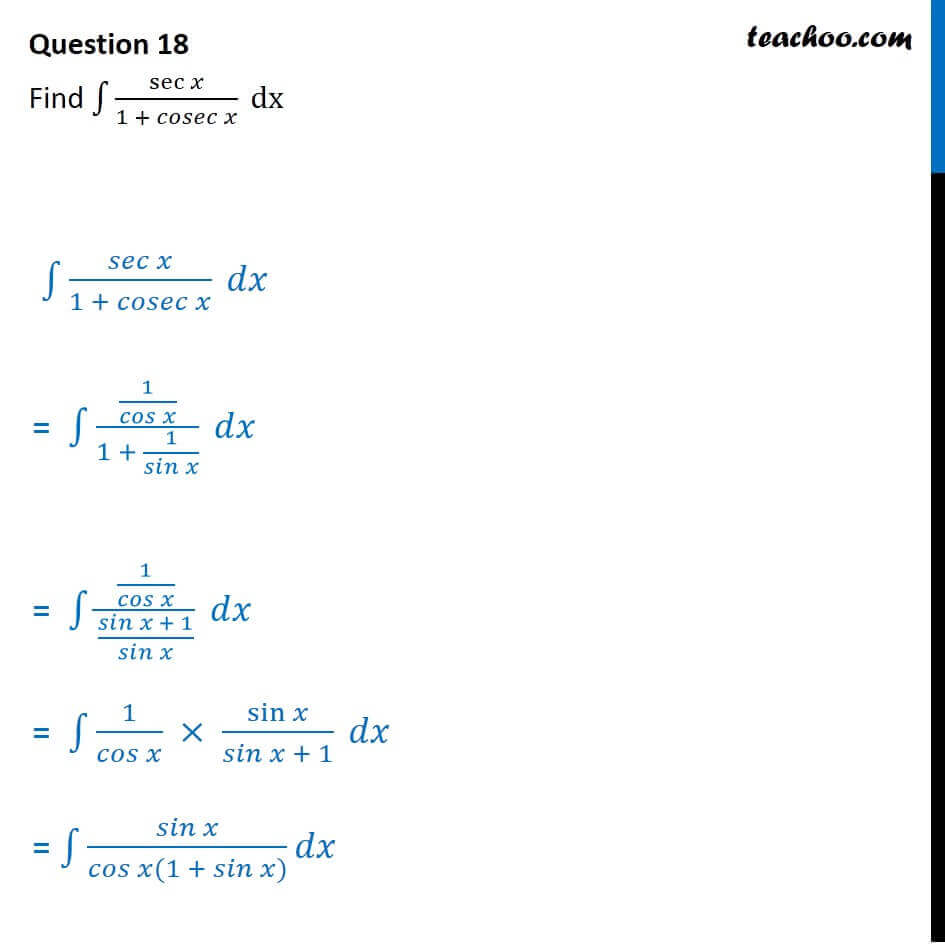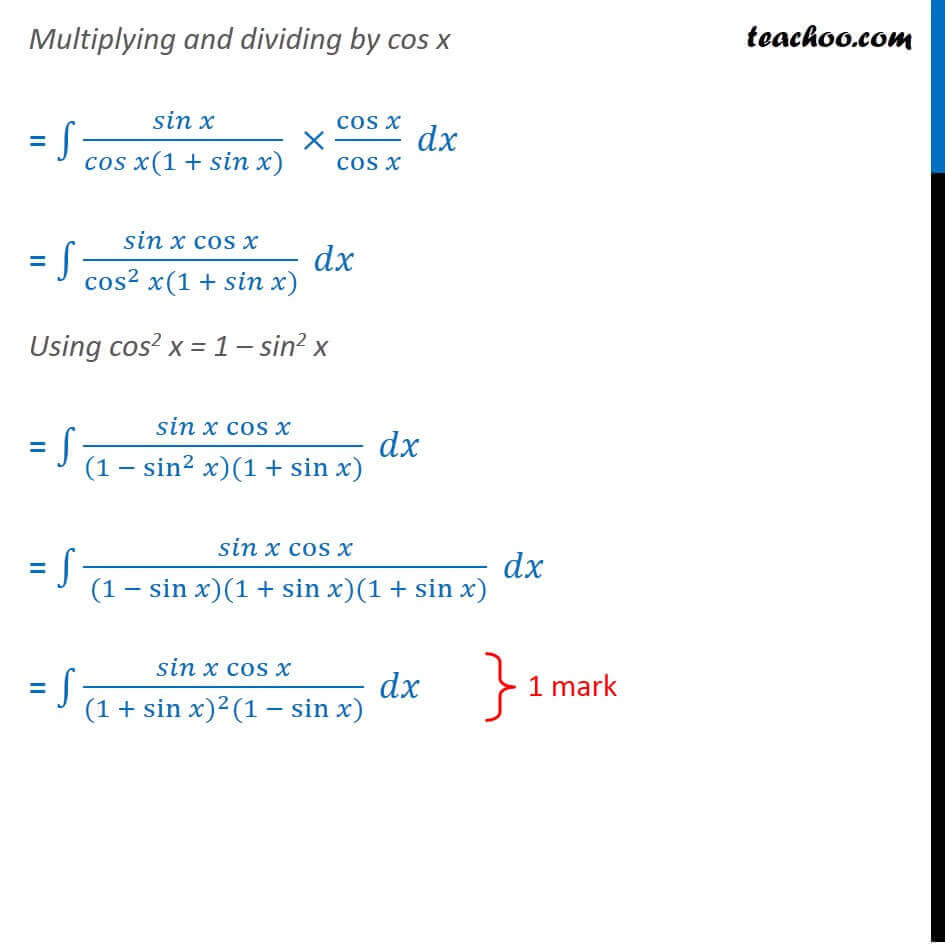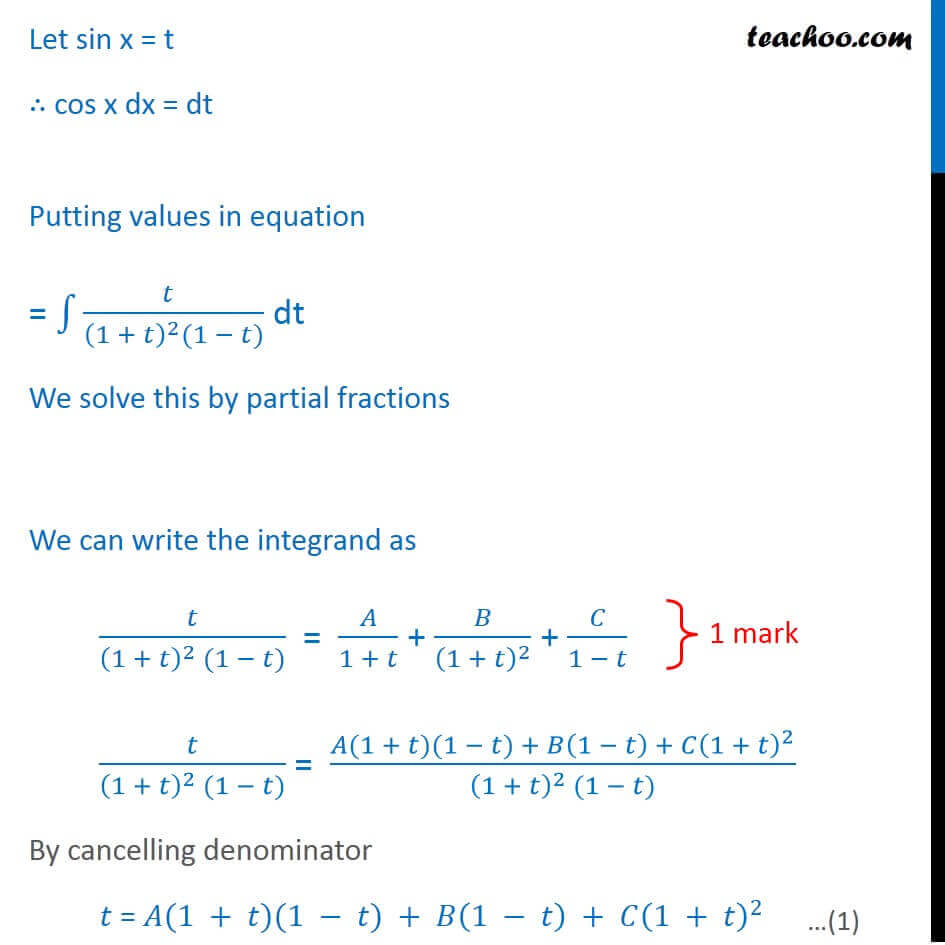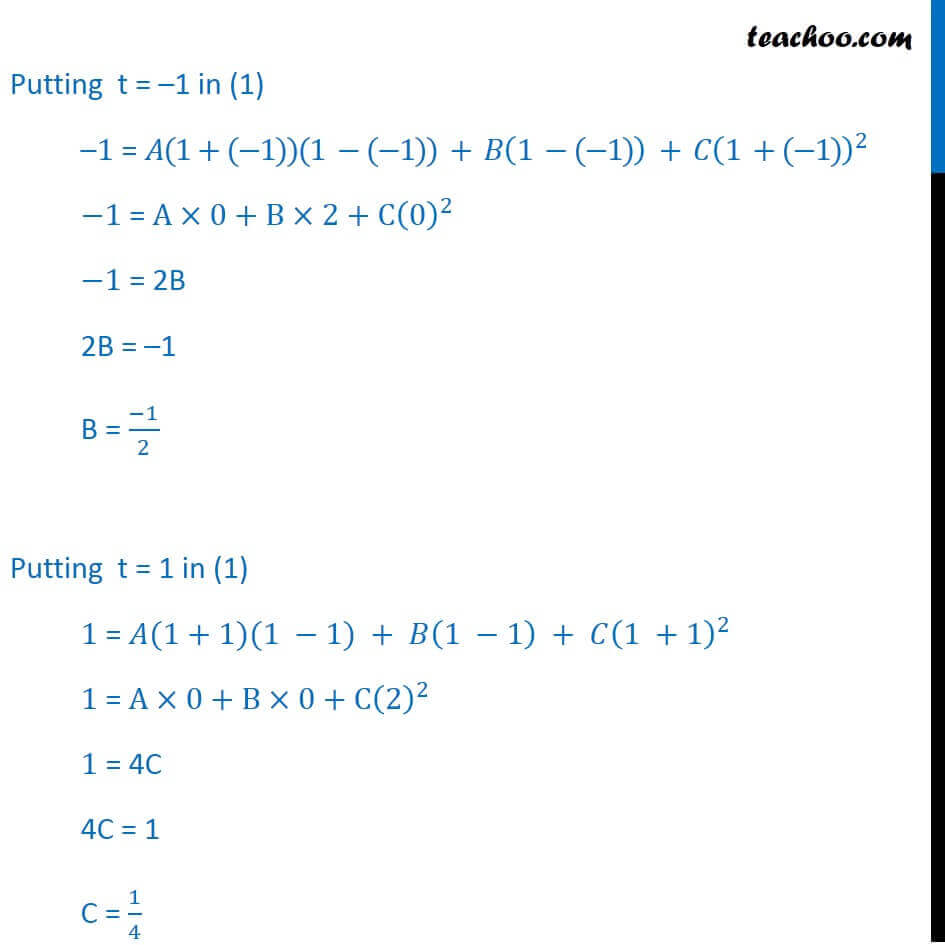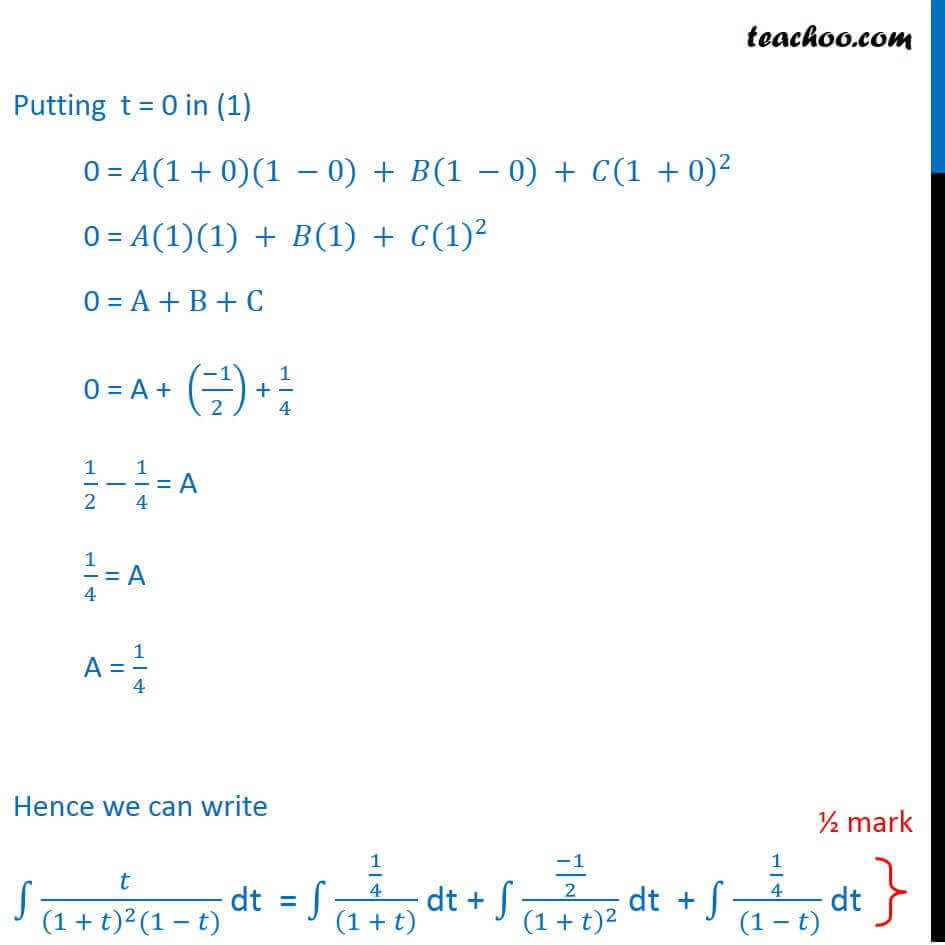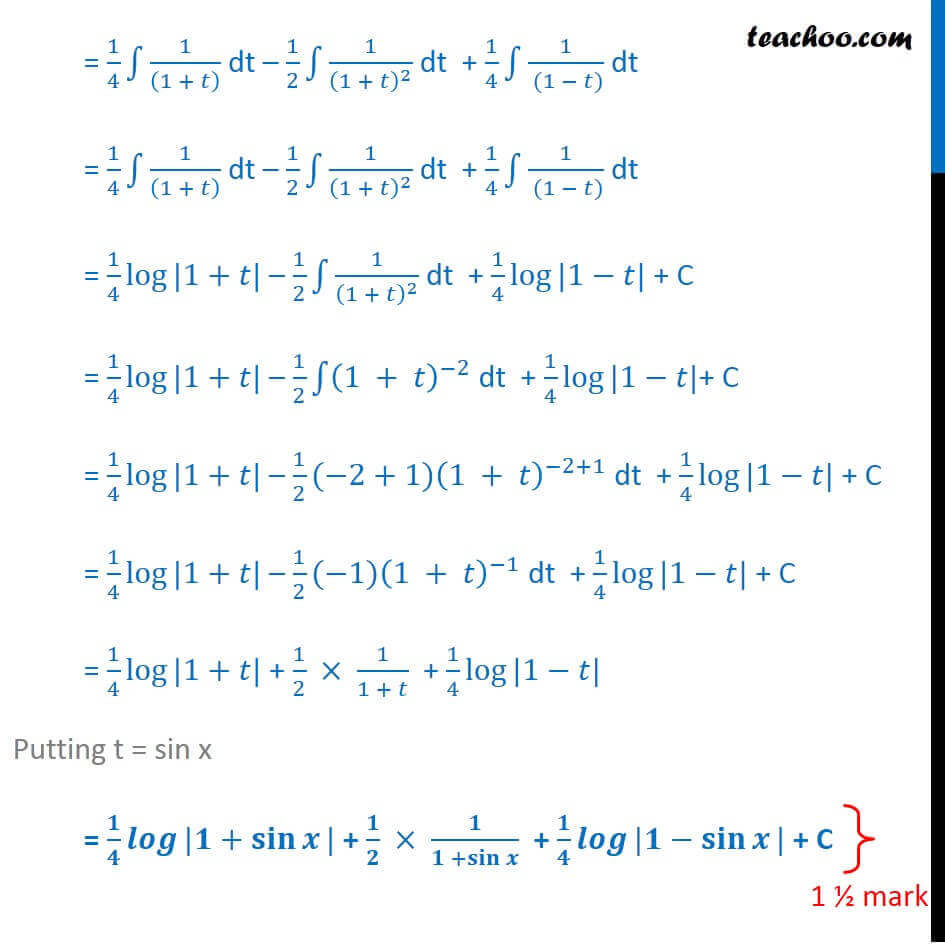Learn in your speed, with individual attention - Teachoo Maths 1-on-1 Class

### Transcript

Question 18 Find ∫1▒sec⁡𝑥/(1 + 𝑐𝑜𝑠𝑒𝑐 𝑥) dx ∫1▒(𝑠𝑒𝑐 𝑥)/(1 + 𝑐𝑜𝑠𝑒𝑐 𝑥) 𝑑𝑥 = ∫1▒(1/𝑐𝑜𝑠⁡𝑥 )/(1 + 1/𝑠𝑖𝑛⁡𝑥 ) 𝑑𝑥 = ∫1▒(1/𝑐𝑜𝑠⁡𝑥 )/((𝑠𝑖𝑛⁡𝑥 + 1)/𝑠𝑖𝑛⁡𝑥 ) 𝑑𝑥 = ∫1▒〖1/𝑐𝑜𝑠⁡𝑥 × sin⁡𝑥/(𝑠𝑖𝑛⁡𝑥 + 1)〗 𝑑𝑥 = ∫1▒(𝑠𝑖𝑛 𝑥)/(𝑐𝑜𝑠 𝑥(1 + 𝑠𝑖𝑛 𝑥)) 𝑑𝑥 Multiplying and dividing by cos x = ∫1▒(𝑠𝑖𝑛 𝑥)/(𝑐𝑜𝑠 𝑥(1 + 𝑠𝑖𝑛 𝑥)) ×cos⁡𝑥/cos⁡𝑥 𝑑𝑥 = ∫1▒(𝑠𝑖𝑛 𝑥 cos⁡𝑥)/(cos^2⁡𝑥 (1 + 𝑠𝑖𝑛 𝑥)) 𝑑𝑥 Using cos2 x = 1 – sin2 x = ∫1▒(𝑠𝑖𝑛 𝑥 cos⁡𝑥)/((1 − sin^2⁡𝑥 )(1 + sin⁡〖𝑥)〗 ) 𝑑𝑥 = ∫1▒(𝑠𝑖𝑛 𝑥 cos⁡𝑥)/( (1 − sin⁡〖𝑥)〗 (1 + sin⁡〖𝑥)〗 (1 + sin⁡〖𝑥)〗 ) 𝑑𝑥 = ∫1▒(𝑠𝑖𝑛 𝑥 cos⁡𝑥)/((1 + 〖sin⁡〖𝑥)〗〗^2 (1 − sin⁡〖𝑥)〗 ) 𝑑𝑥 Let sin x = t ∴ cos x dx = dt Putting values in equation = ∫1▒(𝑡 )/((1 + 𝑡)^2 (1 − 𝑡)) dt We solve this by partial fractions We can write the integrand as 𝑡/((1 + 𝑡)^2 (1 − 𝑡)) = 𝐴/(1 + 𝑡) + 𝐵/(1 + 𝑡)^2 + 𝐶/(1 − 𝑡) 𝑡/((1 + 𝑡)^2 (1 − 𝑡)) = (𝐴(1 + 𝑡)(1 − 𝑡) + 𝐵(1 − 𝑡) + 𝐶(1 + 𝑡)^2)/((1 + 𝑡)^2 (1 − 𝑡)) By cancelling denominator 𝑡 = 𝐴(1 + 𝑡)(1 − 𝑡) + 𝐵(1 − 𝑡) + 𝐶(1 + 𝑡)^2 Putting t = –1 in (1) –1 = 𝐴(1+(−1))(1 −(−1)) + 𝐵(1 −(−1)) + 𝐶(1 +(−1))^2 −1 = A×0+B×2+C(0)^2 −1 = 2B 2B = –1 B = (−1)/2 Putting t = 1 in (1) 1 = 𝐴(1+1)(1 −1) + 𝐵(1 −1) + 𝐶(1 +1)^2 1 = A×0+B×0+C(2)^2 1 = 4C 4C = 1 C = 1/4 Putting t = 0 in (1) 0 = 𝐴(1+0)(1 −0) + 𝐵(1 −0) + 𝐶(1 +0)^2 0 = 𝐴(1)(1) + 𝐵(1) + 𝐶(1)^2 0 = A+B+C 0 = A + ((−1)/2) + 1/4 1/2−1/4 = A 1/4 = A A = 1/4 Hence we can write ∫1▒(𝑡 )/((1 + 𝑡)^2 (1 − 𝑡)) dt = ∫1▒(1/4)/((1 + 𝑡) ) dt + ∫1▒((−1)/2)/(1 + 𝑡)^2 dt + ∫1▒(1/4)/( (1 − 𝑡)) dt